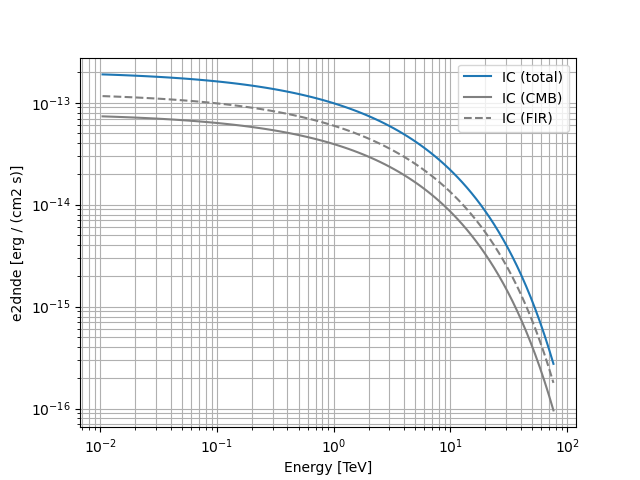# Naima spectral model#

This class provides an interface with the models defined in the naima models module.

The model accepts as a positional argument a Naima radiative `models` instance, used to compute the non-thermal emission from populations of relativistic electrons or protons due to interactions with the ISM or with radiation and magnetic fields.

One of the advantages provided by this class consists in the possibility of performing a maximum likelihood spectral fit of the model’s parameters directly on observations, as opposed to the MCMC fit to flux points featured in Naima. All the parameters defining the parent population of charged particles are stored as `Parameter` and left free by default. In case that the radiative model is `Synchrotron`, the magnetic field strength may also be fitted. Parameters can be freezed/unfreezed before the fit, and maximum/minimum values can be set to limit the parameters space to the physically interesting region.

## Example plot#

Here we create and plot a spectral model that convolves an `ExpCutoffPowerLawSpectralModel` electron distribution with an `InverseCompton` radiative model, in the presence of multiple seed photon fields.

```from astropy import units as u
import matplotlib.pyplot as plt
import naima
from gammapy.modeling.models import Models, NaimaSpectralModel, SkyModel

particle_distribution = naima.models.ExponentialCutoffPowerLaw(
1e30 / u.eV, 10 * u.TeV, 3.0, 30 * u.TeV
)
particle_distribution,
seed_photon_fields=["CMB", ["FIR", 26.5 * u.K, 0.415 * u.eV / u.cm**3]],
Eemin=100 * u.GeV,
)

model = NaimaSpectralModel(radiative_model, distance=1.5 * u.kpc)

opts = {
"energy_bounds": [10 * u.GeV, 80 * u.TeV],
"sed_type": "e2dnde",
}

# Plot the total inverse Compton emission
model.plot(label="IC (total)", **opts)

# Plot the separate contributions from each seed photon field
for seed, ls in zip(["CMB", "FIR"], ["-", "--"]):
model = NaimaSpectralModel(radiative_model, seed=seed, distance=1.5 * u.kpc)
model.plot(label=f"IC ({seed})", ls=ls, color="gray", **opts)

plt.legend(loc="best")
plt.grid(which="both")
```## YAML representation#

Here is an example YAML file using the model:

```model = SkyModel(spectral_model=model, name="naima-model")
models = Models([model])

print(models.to_yaml())
```

Out:

```components:
-   name: naima-model
type: SkyModel
spectral:
type: NaimaSpectralModel
parameters:
-   name: amplitude
value: 1.0e+30
unit: eV-1
error: 0
min: .nan
max: .nan
frozen: false
interp: lin
scale_method: scale10
is_norm: true
-   name: e_0
value: 10.0
unit: TeV
error: 0
min: .nan
max: .nan
frozen: false
interp: lin
scale_method: scale10
is_norm: false
-   name: alpha
value: 3.0
unit: ''
error: 0
min: .nan
max: .nan
frozen: false
interp: lin
scale_method: scale10
is_norm: false
-   name: e_cutoff
value: 30.0
unit: TeV
error: 0
min: .nan
max: .nan
frozen: false
interp: lin
scale_method: scale10
is_norm: false
-   name: beta
value: 1.0
unit: ''
error: 0
min: .nan
max: .nan
frozen: false
interp: lin
scale_method: scale10
is_norm: false
```

Gallery generated by Sphinx-Gallery